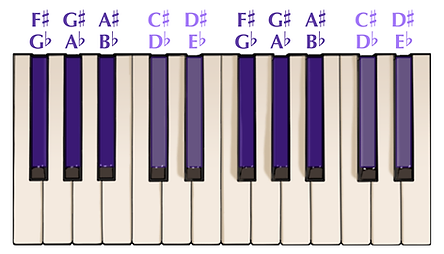top of page

## Understanding Intervals And Accidentals

An interval is the distance from one note to another note either above or below the original note.

Now, the two most basic and most important intervals to know about are a half step and a whole step.

Half Step

A half step is the distance from one note to the very next note either directly above or below the original pitch.

Now, if I was to for instance start on the note B and go up one half step I would end up on the note C. The very next note above the note B is C.Likewise, if I was to start on the note C and go down one half step I would end up on the note B. The very next note below the note C is B.

Let’s try it again this time starting on the note E.

Starting on E and going up one half step would take you to the note F. The very next note directly above the note E is F.And just like earlier if our original note was F and we went down one half step we would end up on the note E. The next note directly below the note F is E.

This is very important to remember. B and C, and E and F, are the only two sets of natural notes which are separated by half steps. All other natural notes are separated by whole steps.

Now that we know what a half step is a whole step will be much easier to understand.

Whole Step

A whole step is the distance of two consecutive half steps.

Let’s start on the note A. If we go up one half step from the note A it will take us to this black key.Now, we know that a half step is two consecutive half steps so if we go up another half step to this black key we will end up on the note, B.Therefore, the notes A and B, having been separated by two consecutive half steps, is separated by one whole step.Let’s try it again starting on the note D.

Just like before, if we start one the note D and go up two half steps we end up on the note E. Two half steps equals one whole step.Now, these black keys do actually half letter names. This is where the term accidentals comes in. An accidental is a symbol placed next to a note that raises or lowers that note by step. Here’s a list of commonly used accidentals.

This here is a sharp. A sharp raises a note by one half step.This means that because this black key is one half step above the note F then this black key is actually the note F-sharp. One half step above the note F is F-sharp.This here is a flat. A flat lowers a note by one half step.If we take a look at the note E and see that this black key is one half step below the note E, then this black key is actually the note E-flat. One half step below E is E-flat.Now, you may be thinking that this black key, E-flat, is also a half step above the note D, and you’re right. Each of the black keys can technically be called two different letter names, one sharp and one flat. Here are the letter names of all the black keys on the piano.bottom of page